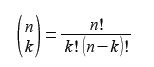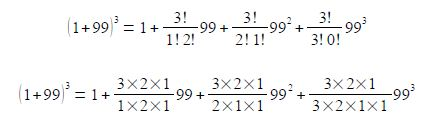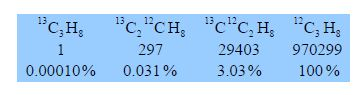Chemistry

# Isotope Abundances of Molecules: Mathematical Example

The binomial formulais used in chemistry for: (a) calculating the intensities of NMR (nuclear magnetic resonance) lines due to spin-spin splitting (Pascal’s triangle);

(b) in mass spectrometry for calculating the ion abundances (intensities) for elements with several isotopes;

(c) to model the biochemical transition between the helix and coiled structures of proteins; and

(d) in statistical mechanics to calculate the probabilities of a given distribution of molecules over a set of quantum states.

You will meet all of these techniques later on in your Chemistry degree.Propane H = gray, C = dark gray

The natural abundance of the stable carbon isotopes are 13C ≈ 1% and 12C ≈ 99%. Calculate the isotopic abundances arising from just the three carbon atoms in propane (ignoring the H-atom isotopes) by expanding the binomial formula (1 + 99)3 into the first 4 terms. These 4 terms correspond to the relative amounts of the following four isotopic molecules Bcgis, 13C3 H8, 13C212 CH8 and 12C3 H8 (these isotopic variants are called isotopomers).

Solution

The shorthand notation used above is called the “binomial bracket”. There is no dividing line and it does not mean n divided by k. It is pronounced “n choose k” and by the binomial theorem it is equal toWhere a fractional is defined as:

n! = n (n-1) + (n-2) + (n-3) … … …1; and by definition, 0! = 1

The carbon atom isotopic abundances in propane are found by expanding the binomial bracket below for the four different isotopomers which “choose” 0, 1, 2, or 3 carbons out of three carbons to be 12C.Expanding the binomial formulae givesCancelling out terms top and bottom within any single term, gives

(1+99)3 = 1+(3X99) + (3X992) + (1X993) = 1+ (297) + (29403) + ( 970299)

In mass spectrometry we normally express the ratios as a percentage of the largest abundance to give the following predicted mass spectrum. Notice how rarely we would find in our mass spectrometer a propane molecule with three 13C atoms (about one in a million propane molecules). On the other hand, just over 3% of the propane molecules contain one 13C atom.